# Excel VBA表單設計，下拉式方塊避免內控輸入錯誤

## Excel VBA的UserForm表單控制項建立下拉式方塊時，設定MatchRequired再配合清單(List)、陣列(Array)、訊息視窗(MsgBox)，可以限定所輸入的資料，避免錯誤，本文以內控測試表單為例介紹。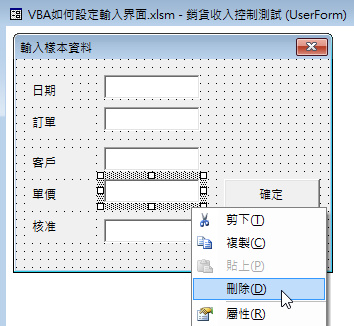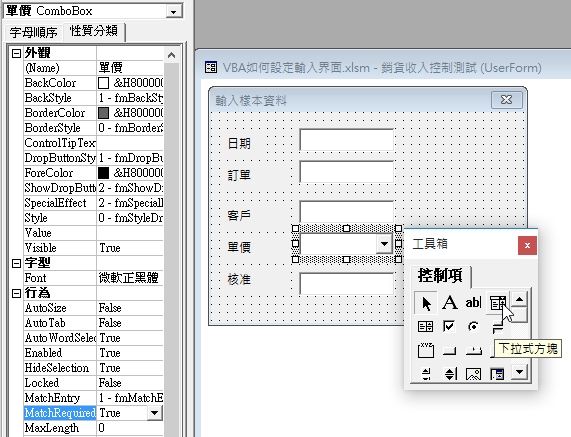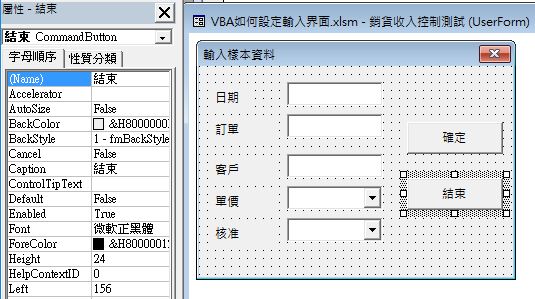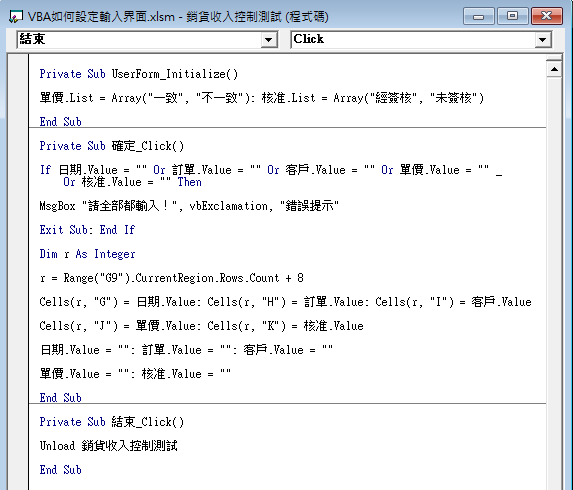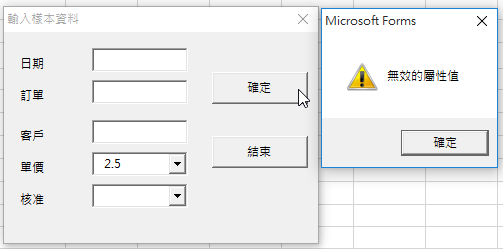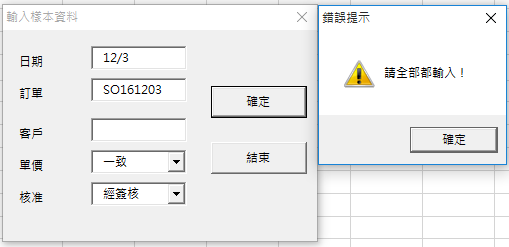Private Sub UserForm_Initialize()

End Sub

Private Sub 確定_Click()

If 日期.Value = “” Or 訂單.Value = “” Or 客戶.Value = “” Or 單價.Value = “” _

Or 核准.Value = “” Then

MsgBox “請全部都輸入！”, vbExclamation, “錯誤提示”

Exit Sub: End If

Dim r As Integer

r = Range(“G9”).CurrentRegion.Rows.Count + 8

Cells(r, “G”) = 日期.Value: Cells(r, “H”) = 訂單.Value: Cells(r, “I”) = 客戶.Value

Cells(r, “J”) = 單價.Value: Cells(r, “K”) = 核准.Value

End Sub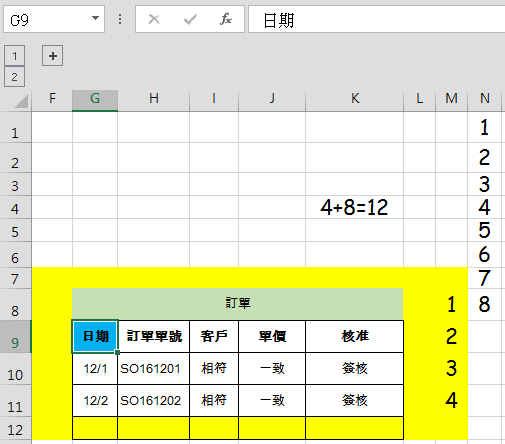Private Sub 結束_Click()

Unload 銷貨收入控制測試

End Sub